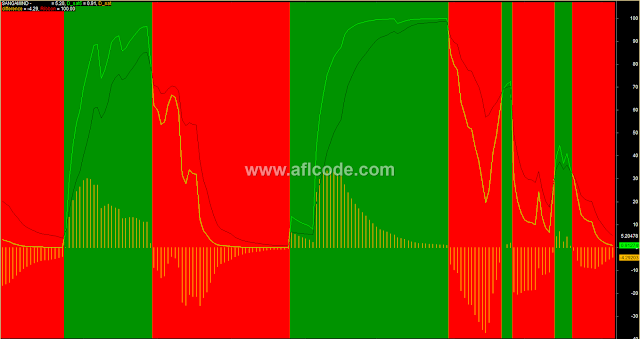### Bullish Bearish Zone Trading SystemBullish Bearish Zone Trading System

```//www.aflcode.com
_SECTION_BEGIN("D_Sat3");

P=30;
CMO30=100*((Sum(IIf(C>Ref(C,-1),(C-Ref(C,-1)),0),P))-(Sum(IIf(C<Ref(C,-1),(Ref(C,-1)-C),0),P)))/((Sum(IIf(C>Ref(C,-1),(C-Ref(C,-1)),0),P)+(Sum(IIf(C<Ref(C,-1),(Ref(C,-1)-C),0),P))));

C1=DEMA(CMO30,30);
D_sat10=RSIa(C1,10);
D_sat5=RSIa(C1,5);
Plot(D_sat10,"D_sat10",1,1);Plot(D_sat5,"D_sat5",5,1);Plot(D_sat5-D_sat10,"D_sat
difference",9,2);

// Rribbon Code starts here

up =Cross(d_sat5,d_sat10)OR d_sat5 > d_sat10;
down =Cross(d_sat10,d_sat5)OR d_sat10 > d_sat5;

//Plot( Close, "Price", colorBlue, styleCandle );
Plot( 100,   /* defines the height of the ribbon in percent of pane
width */
"Ribbon",IIf( up, colorGreen, IIf( down, colorRed, 0 )), /* choose color
*/
styleOwnScale|styleArea|styleNoLabel, -0.5, 100 );
_SECTION_END();
```

Previous
Next Post »